# Relaxing Method in the Evaluation of MPPT of Photovoltaic Cells Based on MIT Modeling

Relaxing Method in the Evaluation of MPPT of Photovoltaic Cells Based on MIT Modeling
College of Engineering and IT, University of Science and Technology, Fujairah POB 2202, U.A.E.

Corresponding Author Email:
a.abdulmajid@ustf.ac.ae
Page:
158-164
|
DOI:
https://doi.org/10.18280/mmep.080120
28 October 2020
|
Accepted:
4 January 2021
|
Published:
28 February 2021
| Citation

OPEN ACCESS

Abstract:

A relaxation method is proposed to determine the maximum power point tracking of a small to medium powered photovoltaic module, based on the non referenced MIT model, with analytical evaluation of the maximum power of the generated voltage. In implementing this algorithm, there is no need for continuous online measurements of temperature or irradiance, which are the most used variables used in the evaluation of the I-V relationship of PV cells. The method has the advantage of adequate accuracy without any oscillations, compared with certain conventional methods. The method can track accurately the maximum power point under changes in atmospheric conditions and load variations, by referring it with the MIT model.

Keywords:

1. Introduction

Renewable energy systems are now widely installed in many places and countries, being competitive sources of electrical power that are free, environment friendly, and with long lifetime. Solar energy systems and particularly photovoltaic (PV) modules are mostly popular due to the ease of converting sun energy to heat and electricity. PV cells are devices that convert solar energy into electricity in a clean way without pollution, that can be used as an alternative solution of fuel power generation in domestic and industrial applications, especially in remote areas. They can be installed as stand alone, grid connected, or as hybrid with other power generating methods.

Although PV modules and panels are easy to be installed their performances are influenced by many factors, such as orientation, weather conditions and their performance in extracting maximum power from solar rays. An overall optimization of the system should be performed to achieve the best possible performance, with the use of measurement equipment, power converters, load and/or grid connection. Several methods have been proposed and studied in the literature to deal with different aspects and cases of solar extraction and control strategies for solar regulators [1, 2].

In contrast to fuel power generating systems, PV modules have rather low power efficiency, and as a result all means are required to maximize this efficiency. One of the most widely implemented technique used to increase efficiency and maximize energy extraction, is the maximum power point tracking (MPPT) method [3, 4], which considers for a certain load, the effect of mainly the solar irradiation and PV cell temperature.

A negative feedback control is employed to track maximum power with the use of output voltage as a reference in a loop to adjust maximum power extracted, using power electronic converters, as depicted in Figure 1, in which the photovoltaic voltage is compared with the maximum power point voltage under standard test conditions (STC), and the error is used to adjust the duty cycle (D) of a regulator or DC/DC converter.

## 1.gifFigure 1. MPP voltage regulator using negative feedback

Many techniques are available in the literature, that propose different MPP methods, in considering effectiveness, range of accuracy, convergence speed, and reliability with the variation of environment conditions such as irradiation and/or temperature change . These techniques differ in many aspects, such as maximum power tracking search algorithm, static or dynamic usage, online or offline connection, arithmetic models or artificial intelligence methods. For instance, online methods need continuous sampling of environment and system whereas offline techniques are simpler, and the operating points are predefined for specific conditions, without system data update .

Search techniques use disturbances in system variables, such as voltage, to find the maximum power point, which is used as the parameter to design the MPP . Among the so many algorithms employed in MPPT problem, the P&O (Perturb and Observe)  and the Incremental Conductance algorithms are the most common . Their main advantages are the easy implementation, low computational demand, and only the measured voltage and current are required as information about the PV array. But they also have some drawbacks, like oscillations around the MPP in steady-state conditions and poor tracking under rapidly changing irradiation levels. This is due to the fact, that the search for maximum power point is related to the choice of the perturbation step. The latter infects the convergence time and the amplitude of the oscillations antagonistically.

Other MPPT algorithms are implemented with fuzzy logic and neural networks, or artificial intelligence (AI) algorithms, using logical and mathematical models , that might require high computational resources, to reach a reliable solution. To overcome these disadvantages and to make the response faster, an optimization technique influenced by the behavior of ant colonies is proposed [11, 12], which requires two control variables, such as input voltage and duty ratio of the converters.

To enhance the performance of the existing methods, several revolutionary computing algorithms are proposed, such as particle Swarm Optimization (PSO), Bacterial Foraging Algorithm (BFA), Ant Colony Optimization (ACO), and Genetic Algorithm (GA) [13, 14]. Other techniques are based on Sliding Mode Control (SMC) method  are used for robust performance to parameter variations, such as nonlinear synergetic controls. Other techniques require look-up tables and curve fitting.

A new and simple implemented optimization algorithm is the Owl Search Algorithm (OSA), which is combined with the P&O technique to track the MPP under steady and non-steady irradiation and temperature conditions .

In this paper, an accurate method is proposed to track the maximum power point of a photovoltaic module. The method is based on a mathematical model of the PV module to compute the theoretical value of the MPP voltage, for an online measured PV current, PV voltage, irradiance, and temperature. The method has the advantage of accuracy without any oscillations, as with some previously mentioned methods. This accuracy depends of course on the accuracy of the model. The MPPT algorithm has also the ability to track, with a fast speed, the maximum power point under variable atmospheric conditions and load changes. Simulation and experimental tests were performed to validate the method.

Whereas model free system uses measurements to obtain the maximum power point, model-based methods require system parameters, such as internal circuit resistance, current or voltage in the STC.

2. PV Model

The PV cell is simply represented as a current source Iph, related with irradiance, in parallel with a diode of fed current of ID related with generated voltage and temperature. It is appropriate to include a small series resistance RS and a larger parallel resistance RP, as shown in Figure 2.

## 2.pngFigure 2. Typical equivalent circuit of a photovoltaic cell, with current-voltage measurements

An ammeter and a voltmeter are connected to measure the output current I and voltage V, of the output load. These values can assist in the process of defining the maximum power point.

As PV cells are connected in series to increase terminal voltage, as well as in parallel to increase supplied current, the formulated equation of the output current is

$I=N_{P} I_{P V}-N_{P} I_{o}\left\{e^{\left[\frac{q\left(V+I R_{S}\right)}{N_{S} K A T_{O}}\right]}-1\right\}-\frac{N_{P}\left(V+I R_{S}\right)}{R_{P}}$    (1)

where, IPV

$I_{P V}=\left[I_{S C}+K_{i}\left(T_{o}-T_{r}\right)\right] S / S_{r}$      (2)

where, NS and NP are the number of series and parallel connected cells respectively.

It can be noted that to find the I-V relationship, both temperature T and irradiance S are to be included. Consequently, it is required to measure all four parameters: I, V, T, and S. The series resistance RS is normally very small, while the parallel resistance RP is large, hence it is possible to simplify the equivalent circuit by ignoring both of them.

Figure 3 demonstrates the effect of irradiance and operating cell temperature on the I-V relationship. In essence, current is linearly proportional with solar irradiance, and inversely proportional with temperature, while the effect is minor on the voltage. It can be noticed that the slopes of dV/dI and dI/dV are same for any irradiance or temperature.

## 3.png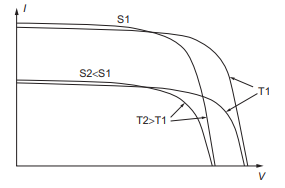Figure 3. Irradiance and temperature effects on I-V relationship

2.1 MIT model

The equivalent circuit of a PV cell has been simulated in several expressions, depending on complexity, accuracy and variables used. To find an expression under nonreferenced irradiance and operational temperature, the MIT model is employed, also named the Jet Propulsion Lab (JPL). It is a useful model for our proposed relaxation method in determining the MPP. In this model, the I-V depends only on ISC, IMP, VMP and VOC, as

$I=I_{S C}-I_{S C} C_{1} \exp \left(\frac{V}{C_{2} V_{O C}}-1\right)$     (3)

where,

$C_{1}=\left(1-\frac{I_{M P}}{I_{S C}}\right) \exp \left(\frac{V_{M P}}{C_{2} V_{O C}}\right)$      (4)

$C_{2}=\left(\frac{V_{M P}}{V_{O C}}-1\right) / \ln \left(1-\frac{I_{M P}}{I_{S C}}\right)$       (5)

In fact, IMP, VMP can be determined from ISC and VOC too. Figure 3 depicts the I-V relation for general non referenced conditions.

The characteristic can be extended to referenced conditions of irradiance and temperature, as well as the shunt and series resistances representing the detailed equivalent circuit of a PV cell. In general, current is proportional to irradiance, whereas voltage is related to temperature, hence both of them can be related to the referenced values as

$I_{S C}=I_{S C R} S$      (6)

and

$V_{O C}=V_{O C R}-0.0023 T_{A}(T-25)$      (7)

where, ISCR is the referenced short circuit at 1kW/m2, S is operational irradiance in kW/m2, VOCR is referenced open circuit voltage at 250C, TA is the ambient temperature and T is the solar cell temperature, which is defines as

$T=T_{A}+\frac{(N O C T-20) S}{0.8}$     (8)

NOCT is the normal operating cell temperature of the PV cell when operating under the conditions of S=0.8kW/m2, and ambient temperature of 20℃. Thereafter the maximum power can be calculated as

$P_{M P}=I_{S C} V_{O C} F F$      (9)

where, FF is the fill factor defined as PMPR/(ISCR VOCR), with PMPR is the referenced maximum power, declared by the manufacturer.

The most basic and important electrical characteristics of PV cells are the open-circuit voltage, short circuit current and maximum power point, which are affected by both irradiance and operational temperature. The temperature coefficient for the open-circuit voltage of a PV cell is around -2.2mV/℃, and can be accumulated with the use of more cells connected in series, whereas the current coefficient is about 5uA/℃,/cm2, which can be neglected. On the other hand, short-circuit current is proportional to the daily irradiance, and can be related to 1 kW/m2 reference. Since the voltage is a logarithmic function of current, it will also vary logarithmically with irradiance.

Figure 4 depicts the voltage and current dependencies on irradiance at a reference operating temperature of 250C, whereas Figure 5 depicts the variations of voltage and current with temperature at a constant solar irradiance of 1000W/Sq. m. These figures are based on the MIT model that used as a reference platform for other environmental conditions of irradiance and temperature.

The difference between cell temperature and ambient temperature is linearly dependent on irradiance as well, with factor $[N O C T-20] S / 0.8$, where NOCT stands for Normal Operating Cell temperature, and S is irradiance in kW/m2. In order to simplify all variations, it is customary to neglect both voltage variation due to irradiance and current variation due to operational temperature.

## 4.pngFigure 4. Variations of I-V with irradiance at constant temperature

## 5.pngFigure 5. I-V variations with temperature at constant irradiance

3. MPPT Methods

The output dc power of a PV cell or module is V x I, which is variable with both of them, reaching a maximum of PMP, and in order to extract maximum power, we need to operate dynamically on load at the maximum power point MPP. That is when dP/dV=0. It is noted that this point depends on continuous variations of the output load, as well as with irradiation and operational temperature. This is mostly required especially for PV modules power in excess of 1kW.

For lower output powers, a simpler yet efficient algorithm is needed to trace MPP.

3.1 Relaxation method

The I-V characteristic of a PV cell has the same pattern for any variations in environmental irradiation or operational temperature. The current varies almost linearly with small slope before the maximum power point MPP, and also in a linear matter with large slope after the MPP point. In almost all cases, the MPP lies between these two regions.

Figure 6 demonstrates a general I-V pattern of a PV cell with two slope lines, that’s ΔI/ ΔV, at the short circuit current ISC and open circuit voltage VOC, in which the former represents the series resistance RS, while the latter represents the shunt resistance RSH,

## 6.pngFigure 6. Effects of RS and RP on I-V curve pattern

The maximum power point is normally determined from the power-voltage characteristic as depicted in Figure 7. As seen, the three shown slopes represent the conductance G=1/V, at different curve locations with maximum power point located at G=0. It can be noted that MPP voltage can also be approximated from the intersection of G>0 and G<0, yet this has not been investigated in this study.

## 7.pngFigure 7. Power-voltage relationship

## 8.png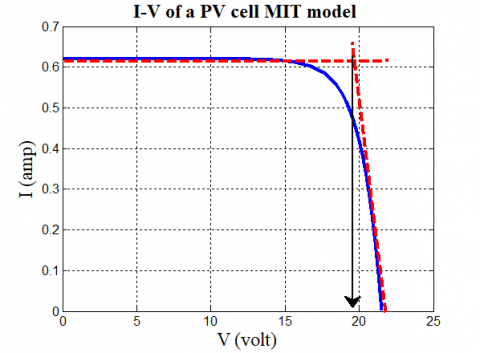Figure 8. Slope method in the I-V relationship

The slope method of determining MPP voltage, is used in the I-V relationship, as shown in Figure 8 to give reasonably accurate value for small and medium module ratings within 1kW. It can be seen that using MIT model, the MPP voltage of 19.9 V is determined, compared with 19.6 V as reported by module data.

It can also be seen that this same MPP voltage can be determined with the P-V relationship with a similar accuracy, as depicted in Figure 9.

## 9.pngFigure 9. Slope method in the P-V relationship

We shall implement the method on the I-V relationship, since they are readily available for the implemented mathematical model. It must be emphasized that these relationships depend on irradiance and operating voltage, and the method can only be applied when these environmental effects are taken into consideration.

## 10.png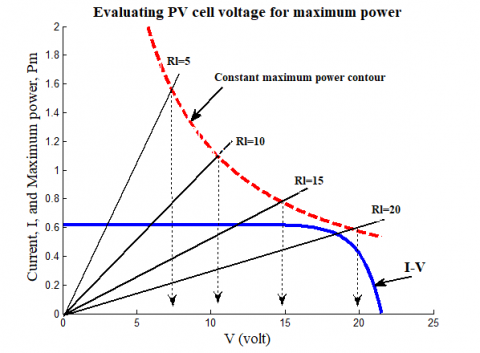Figure 10. Estimating MPP voltage for different load resistances

The load resistance determines the MPP for fixed values of irradiance and operating temperature. Figure 10 demonstrates different values of load resistances ranging from 5-20 Ω, that are drawn in the I-V for the PV module studied in this work. The maximum power point power is calculated as a constant value of $\operatorname{Imp} x \mathrm{Vmp}$ and drawn for different values of voltages and currents as shown. The load resistance lines are intersecting this constant maximum power curve at different values of voltages, with smaller voltages at lower resistances. These voltages represent the controlled output voltages needed to operate at MPP region, as feedback values to be compared with PV measured voltages used as references for the feedback, as shown in Figure 1. Here, the curve scale of constant maximum power is only representative, yet it will be evaluated in simulating a case study.

Analytically, it can be deduced that the equations of the two slopes lines of the I-V relationship at Voc and Isc are

$V_{m}=\left(I_{s c} R_{s}-V_{o c}\right) /\left(\frac{R_{s}}{R_{p}}-1\right)$     (10)

$I_{m}=I_{s c}-V_{m} / R_{p}$      (11)

and the intersection of these two lines is the MPP voltage, with maximum power evaluated as $V_{m} \times I_{m}$, hence the MPP output controlled voltage can be determined at any load resistance as the projection at the constant power curve intersections, as demonstrated in Figure 10, that’s

$V_{o}=\sqrt{V_{m} I_{m} R_{L}}$      (12)

It can be noted that the controlled output voltages V0 is smaller at lower values of load resistances, than at larger load resistances. A Buck-Boost converter is normally used to bring the measured PV cell voltage VPC to the MPP controlled voltage Vo with controlled duty cycle (D) evaluated

$D=\left(V_{o}-V_{P C}\right) / V_{o}$      (13)

In this method, tracking MPP with voltage perturbations, is carried out once without the continuous variations of voltage and power monitoring as for example in the P&O method, and other methods, with eliminated oscillations of tracking.

4. Simulation and Case Studies

4.1 MIT model simulation

## 11.pngFigure 11. Variation of voltage with irradiance and temperature

The accuracy of the MIT model of simulating the PV cell requires evaluation of voltage and current variation with the two main effects, that are temperature and irradiance. Figure 11 depicts the variation of PV cell voltage with respect to both temperature and irradiance.

It can be noted that the effect of temperature in minimum, whereas voltage is linearly proportional with irradiance.

Similarly, Figure 12 shows a surface of current variation with temperature and irradiance.

## 12.png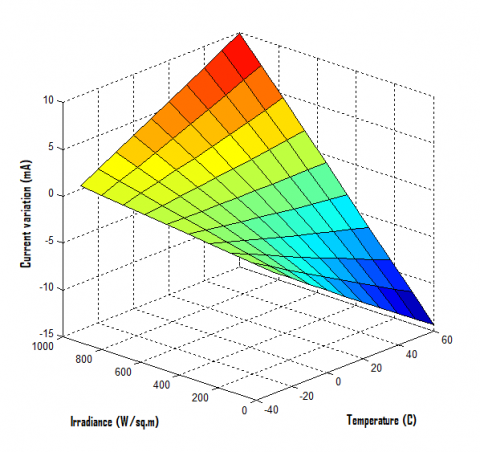Figure 12. Variation of current with irradiance and temperature

Apart from temperature and irradiance effects on working at maximum power point, the variation of the load resistance changes MPP location. Figure 13 demonstrates the effect of load resistance changes on controlling the output voltage on the output load, which can be a battery for the case of standalone setup or connected with the ac grid network.

## 13.png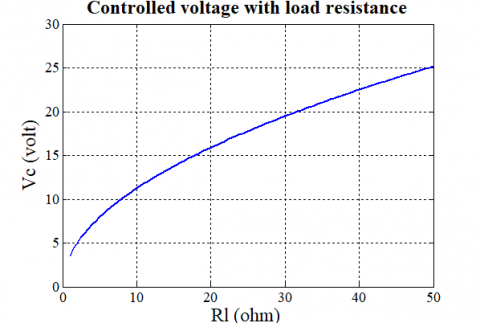Figure 13. Output controlled voltage with different load resistances

4.2 MPP controlled voltage simulations

The case studies simulations are based on the data of a PV module that was used for its equivalent circuit represented in the MIT model, as indicated in Table 1. The method of finding MPP is demonstrated with variations of temperature in the range of 10℃-40℃, with variations in irradiance in the range of 200W/m2-1000W/m2, as well as 3 variations in load resistance of 10, 30 and 50 Ω. The 30 Ω resistance represents approximately the characteristic resistance of the PV cell, as per following studied module data, since it is equal to $V m p / I m p=16.9 / 0.6=28.167 \Omega$.

Table 1. PV module data used in study

 Name Symbol value Module power Pmp 10 W MMP voltage Vmp 16.9 V MMP current Imp 0.6 A Open circuit voltage Voc 21.5 V Short circuit current Isc 0.62 A Series resistance Rs 1.72 Ω Parallel resistance Rp 28 K Ω Current coefficient α 0.27 mA/0C Voltage coefficient β -82.4 mV/0C

Under these different values of irradiance and operating temperature, the MPP controlled voltage is evaluated for different values of load resistances, as depicted in Table 2.

Table 2. MPP controlled voltage for variations in irradiance, temperature and load resistance

 Temperature Irradiance S, W/m2 (MPP) controlled voltage Vo Rl=10 Ω Rl=30 Ω Rl=50 Ω 25 0C 200 11.3375 19.6367 25.3509 25 0C 500 11.4823 19.8879 25.6752 25 0C 1000 11.7395 20.3335 26.2504 10 0C 200 10.9886 19.0329 24.5713 10 0C 500 11.1275 19.2735 24.8819 10 0C 1000 11.3731 19.6988 25.4311 40 0C 200 11.6763 20.2239 26.1090 40 0C 500 11.8284 20.4875 26.4492 40 0C 1000 12.0989 20.9559 27.0540

In order to verify the proposed MIT method in evaluating maximum power point, a load resistance of 30 Ω is selected to be similar to the characteristic resistance of the adopted PV module in this study. Table 3 depicts the determined controlled voltage V0 to evaluate maximum power point voltage (Vmp), with a controlled converter duty cycle (D). Maximum current and power are also evaluated at the MPP.

Table 3. Duty cycle values used to control output voltage for MPP volatge

 S W/m2 MIT Model Duty cycle Vo Vmp Imp Pmp D % 200 19.6367 20.4366 0.6188 12.6456 -4.0735 500 19.8879 20.4360 0.6190 12.6491 -2.7559 1000 20.3335 20.4350 0.6193 12.6548 -0.4992 200 19.0329 19.2033 0.6180 11.8678 -0.8953 500 19.2735 19.2069 0.6170 11.8503 0.3456 1000 19.6988 19.2129 0.6153 11.8210 2.4666 200 20.2239 21.6699 0.6195 13.4254 -7.1500 500 20.4875 21.6651 0.6209 13.4528 -5.7479 1000 20.9559 21.6572 0.6233 13.4984 -3.3466

Note: A load resistance of 30 Ω is used. The first blue highlighted 3 values are for temperature of 25℃, the second green highlighted 3 values are for 10℃, and the third orange 3 values are for 40℃.

4.3 Case study

The evaluation of MMP controlled voltage is demonstrated in a general case study according to variations of irradiance (S) and operating temperature (T) as well as load resistance (RL), as depicted in Figure 14, in which three time incidents during daily sun path, are selected to implement the employed method in selecting MPP voltages.

## 14.pngFigure 14. MPP method case study

The MPP controlled voltage as well as voltage and current perturbations are tabulated in Table 4, for different values of irradiance (S), temperature (T) and load resistances (R), at different instants of time. It must be noted that variations of load resistance is normally at higher pace than both irradiance and temperature variations during the sun path 6 AM- 6 PM. These parameters are selected to correspond with the data of the PV module that is implemented in this study.

Table 4. MPP voltage with current and voltage vairations at three different time incidents

 S W/m2 T 0C R Ω MPP Voltage dV dI Time 1 800 20 10 20.4354 0.3445 -0.1237 Time 2 400 30 5 20.4362 0.7056 -0.3719 Time 3 700 25 15 20.4356 0.4348 -0.1857
5. Conclusions

A relaxation algorithm is proposed for small and medium sized PV modules, in which the I-V pattern at reference values, is reduced to two linear slopes, intersecting at the maximum power point. The slopes depend on the series and parallel resistances of the equivalent PV cell circuit. The controlled voltage to be used in the Buck-Boost converter, depends on the evaluated maximum power and load resistance.

The well-known MIT or JPL non-referenced method of analyzing the equivalent circuit is implemented, that doesn’t depend on continuous measurements of irradiance and operating temperature. The entire analysis is mathematical and requires one V-I measurements per load resistance value, and as irradiance and temperature vary, the MIT model is adjusted according to mathematical formulations.

A simulation case study is used to evaluate the duty cycle of a voltage converter, and to compare the used MIT method with the data of an implemented PV module.

Nomenclature
 NP number of parallel cells in module IPV PV cell current, A Io diode reverse (dark) current, A q electron charge, columb V Measured cell output voltage I Measured cell output current RS Series resistance NS Number of series cells in module K Boltzmann constant A Efficiency constant To Cell operating temperature RP Parallel resistance ISC Short circuit current Ki Constant Tr Reference temperature S Irradiance Sr Reference irradiance C1, C2 Symbols used in MIT equations VOC Open circuit voltage VMP Maximum power point voltage IMP Maximum power point current ISCR Short circuit current for a referenced value VOCR Open circuit voltage for a referenced value TA Ambient temperature NOCT Normal Operating Cell Temperature
References

 Hodge, B.K. (2010). Photovoltaic systems. Alternative Energy Systems and Applications, A book published by John Wiley & Sons, 225-248. ISBN:978-0-470-14250-9

 Freris, L. Infield, D. (2008). Electrical power generation and conditioning. Renewable Energy in Power Systems, book published by WILEY, 97–147. ISBN: 978-0-470-01749-4

 Markvart, T. (2000). Photovoltaic system engineering. Solar Electricity. John Wiley & Sons, 82-136. ISBN:0-471-98853-7.

 Bhatnagar, P., Nema, R.K. (2013). Maximum power point tracking control techniques: State-of-the-art in photovoltaic applications. Renewable and Sustainable Energy Reviews, 23: 224-241. https://doi.org/10.1016/j.rser.2013.02.011.

 Villalva, M.G., Gazoli, J.R., Ruppert Filho, E. (2009). Comprehensive approach to modeling and simulation of photovoltaic arrays. IEEE Transactions on Power Electronics, 24(5): 1198-1208. https://doi.org/10.1109/TPEL.2009.2013862

 Hohm, D.P., Ropp, M.E. (2003). Comparative study of maximum power point tracking algorithms. Progress in Photovoltaics: Research and Applications, 11(1): 47-62. https://doi.org/10.1002/pip.459

 El Fadili, A., Giri, F., El Magri, A. (2013). Reference voltage optimizer for maximum power tracking in single-phase grid-connected photovoltaic systems. Journal of Control and Systems Engineering, 1(2): 57.

 Petrone, G., Spagnuolo, G., Teodorescu, R., Veerachary, M., Vitelli, M. (2008). Reliability issues in photovoltaic power processing systems. IEEE transactions on Industrial Electronics, 55(7): 2569-2580. https://doi.org/10.1109/TIE.2008.924016

 Qin, S., Wang, M., Chen, T., Yao, X. (2011). Comparative analysis of incremental conductance and perturb-and-observation methods to implement MPPT in photovoltaic system. In 2011 International Conference on Electrical and Control Engineering, 5792-5795. https://doi.org/10.1109/ICECENG.2011.6057704

 El Jouni, A., El Bachtiri, R. (2008). A maximum power point tracking fuzzy logic controller for photovoltaic pumping system. International Scientific Journal for Alternative Energy and Ecology, 5(61): 51-57.

 El Jouni, A., El-Bachtiri, R., Boumhidi, J. (2006). Sliding mode controller for the maximum power point tracking of a photovoltaic pumping system. WSEAS Transactions on Power Systems, 1(10): 1675.

 Dorigo, M., Gambardella, L.M. (1997). Ant colony System: Optimization by a colony of cooperating agents. IEEE Transactions on Systems, Man and Cybernetics, (Part B), 29-41.

 Miyatake, M., Veerachary, M., Toriumi, F., Fujii, N., Ko, H. (2011). Maximum power point tracking of multiple photovoltaic arrays: A PSO approach. IEEE Transactions on Aerospace and Electronic Systems, 47(1): 367-380. https://doi.org/10.1109/TAES.2011.5705681

 Borni, A., Abdelkrim, T., Bouarroudj, N., Bouchakour, A., Zaghba, L., Lakhdari, A., Zarour, L. (2017). Optimized MPPT controllers using GA for grid connected photovoltaic systems, comparative study. Energy Procedia, 119: 278-296. https://doi.org/10.1016/j.egypro.2017.07.084

  Mehiri, A., Bettayeb, M., Hamid, A.K. (2019). Fractional nonlinear synergetic control based MPPT Algorithm for PV System. In 2019 Advances in Science and Engineering Technology International Conferences (ASET), pp. 1-5. https://doi.org/10.1109/ICASET.2019.8714527

 Jain, M., Maurya, S., Rani, A., Singh, V. (2018). Owl search algorithm: A novel nature-inspired heuristic paradigm for global optimization. Journal of Intelligent & Fuzzy Systems, 34(3): 1573-1582. https://doi.org/10.3233/JIFS-169452